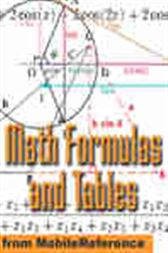Math Formulas and TablesUS\$19.99
ISBNs
• 1605010812
• 9781605010816
An essential reference guide for Math, Science, and Engineering students. You will use it from high school all the way to graduate school and beyond. FREE Functions, Equations, and Table of Derivatives in the trial version.

Algebraic formulas
Trigonometric formulas
Geometric formulas
Linear Algebra formulas
Calculus formulas
Statistical formulas
Table of Derivatives
Table of Integrals
Table of Mathematical Symbols
Access the guide anytime, anywhere - at home, on the train, in the subway.
Always have the guide available for a quick reference.

• MobileReference.com; January 2007
• ISBN: 9781605010816